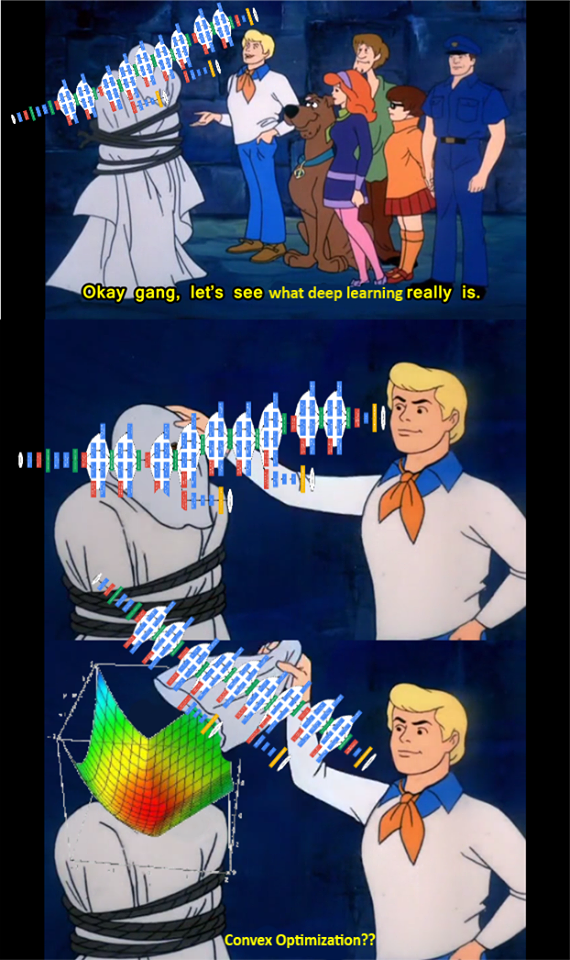# ProblemA lot of practical task nowadays are being solved by the deep learning approach, which is usually implies finding local minimum of a non - convex function, that generalizes well (enough 😉). The goal of this short text is to provide you an importance of the optimization behind neural network training.

## Cross entropy

One of the most commonly used loss functions in classification tasks is the normalized categorical cross entropy in $K$ class problem:

Since in Deep Learning tasks the number of points in a dataset could be really huge, we usually use Stochastic gradient descent based approaches as a workhorse.

In such algorithms one uses the estimation of a gradient at each step instead of the full gradient vector, for example, in cross entropy we have:

The simplest approximation is statistically judged unbiased estimation of a gradient:

where we initially sample randomly only $b \ll n$ points and calculate sample average. It can be also considered as a noisy version of the full gradient approach.# Code